# CS GATE 2013 - Online Test

Q1. Determine the maximum length of cable (in km) for transmitting data at a rate of 500 Mbps in an Ethernet LAN with frames of size 10,000 bits. Assume the signal speed in the cable to be 2,00,000 km/s
Answer : Option B
Explaination / Solution:Q2. A scheduling algorithm assigns priority proportional to the waiting time of a process. Every process starts with priority zero(the lowest priority). The scheduler re-evaluates the process priorities every T time units and decides the next process to schedule. Which one of the following is TRUE if the processes have no I/O operations and all arrive at time zero?
Answer : Option B
Explaination / Solution:

The given scheduling definition takes two parameters, one is dynamically assigned process priority and the other is ‘T’ time unit to re-evaluate the process priorities. This dynamically assigned priority will be deciding processes order in ready queue of round robin algorithm whose time quantum is same as ‘T’ time units. As all the processes are arriving at the same time, they will be given same priority but soon after first ‘T’ time burst remaining processes will get higher priorities

Q3. A binary operation ⊕ on a set of integers is defined as x ⊕ y = x2 + y2. Which one of the following statements is TRUE about ⊕?
Answer : Option A
Explaination / Solution:

x ⊕ y = x2 + yyx2 = y ⊕ x
∴ commutative
Not associative, since, for example
(1 ⊕ 2) ⊕ 3 ≠ 1 ⊕ (2 ⊕ 3)

Q4. A RAM chip has a capacity of 1024 words of 8 bits each (1K × 8) . The number of 2 × 4 decoders with enable line needed to construct a 16K × 16 RAM from 1K × 8 RAM is
Answer : Option B
Explaination / Solution:

RAM chip size = 1k × 8 [1024 words of 8 bits each]
RAM to construct = 16k × 16
Number of chips required = (16k × 16)/(1k × 8) = 16 × 2 [16 chips vertically with each having 2 chips horizontally]
So to select one chip out of 16 vertical chips, we need 4 x 16 decoder.
Available decoder is – 2 x 4 decoder
To be constructed is 4 x 16 decoder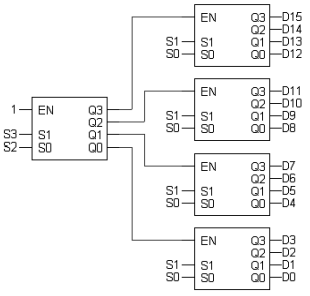So we need 5, 2 x 4 decoder in total to construct 4 x 16 decoder.

Q5.  Consider the following sequence of micro–operations
MBR ← PC
MAR ← X
PC ← Y
Memory ← MBR
Which one of the following is a possible operation performed by this sequence?
Answer : Option D
Explaination / Solution:

PC content is stored in memory via MBR and PC gets new address from Y. It represents a function call (routine), which is matching with interrupt service initiation

Q6. Which one of the following is NOT logically equivalent to  ¬∃x (∀y (α) ∧ ∀z (β))?
Answer : Option A
Explaination / Solution:
No Explaination.

Q7. Consider an instruction pipeline with five stages without any branch prediction: Fetch Instruction (FI), Decode Instruction (DI), Fetch Operand (FO), Execute Instruction (EI) and Write Operand (WO). The stage delays for FI, DI, FO, EI and WO are 5 ns, 7 ns, 10 ns, 8 ns and 6 ns, respectively. There are intermediate storage buffers after each stage and the delay of each buffer is 1 ns. A program consisting of 12 instructions I1, I2 ,I3 ,......I12 is executed in this pipelined processor. Instruction I4 is the only branch instruction and its branch target is I9 . If the branch is taken during the execution of this program, the time (in ns) needed to complete the program is
Answer : Option B
Explaination / Solution:

Clock period=Maximum stage delay+ overhead (Buffer) =10+1=11 ns
Assume FI-1, DI-2, FO-3, EI-4, WO-5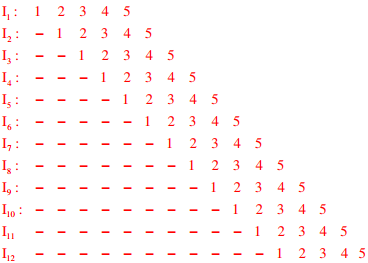So number of clocks required to complete the program is = 15 clocks and time taken is = 15 ×11 ns=165 ns.

Q8. The following figure represents access graphs of two modules M1 and M2. The filled circles represent methods and the unfilled circles represent attributes. IF method m is moved to module M2 keeping the attributes where they are, what can we say about the average cohesion and coupling between modules in the system of two modules?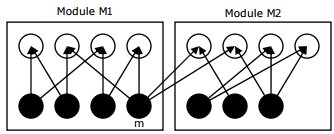Answer : Option A
Explaination / Solution: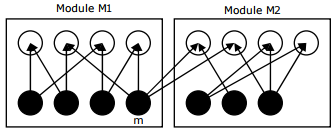Coupling = number of external links/number of modules = 2/2
Cohesion of a module = number of internal links/number of methods
Cohesion of M1 = 8/4; Cohesion of M2 = 6/3; Average cohesion=2
After moving method m to M2, graph will become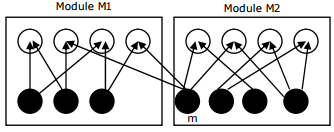Coupling = 2/2
Cohesion of M1 = 6/3; Cohesion of M2 = 8/4; Average cohesion=2

Q9. An index is clustered, if
Answer : Option C
Explaination / Solution:

Clustered index is built on ordering non key field and hence if the index is clustered then the data records of the file are organized in the same order as the data entries of the index.

Q10. Consider a hard disk with 16 recording surfaces (0 - 15) having 16384 cylinders (0 - 16383) and each cylinder contains 64 sectors (0 - 63) . Data storage capacity in each sector is 512 bytes. Data are organized cylinder–wise and the addressing format is . A file of size 42797 KB is stored in the disk and the starting disk location of the file is <1200, 9, 40>. What is the cylinder number of the last sector of the file, if it is stored in a contiguous manner?
Answer : Option D
Explaination / Solution:

42797 KB = (42797 × 1024)/512 = 85594 sectors
Starting is (1200,9,40) contains total 24 + (6×64)=408 sectors
Next, 1201, --------, 1283 cylinders contains total 1024×83 = 84992 sec tors
each cylinder contains 16×64 = 1024 sec tors)
Total = 408+84992 = 85400 sec tors
The required cylinder number is (1284) which will contain the last sector of the file